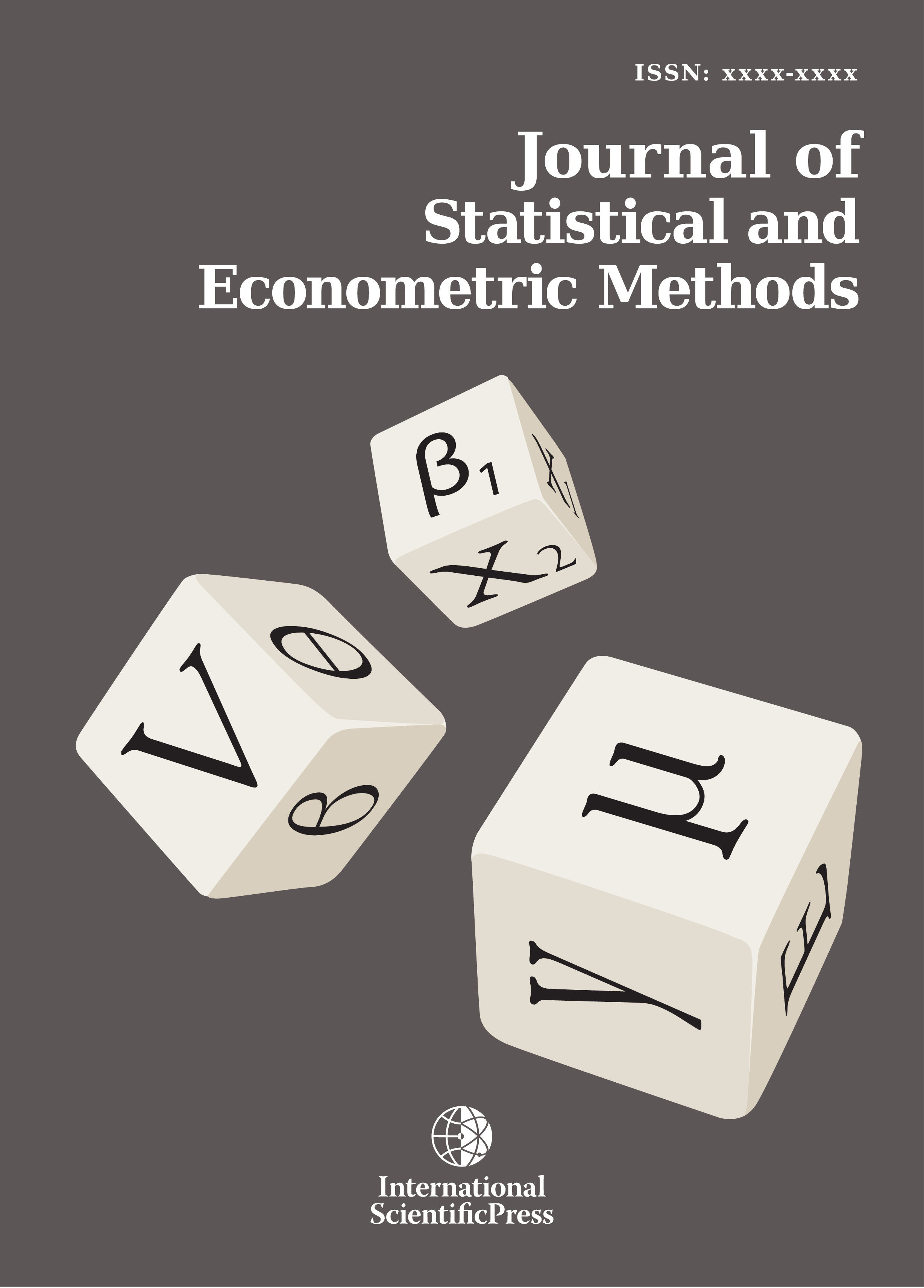# Journal of Statistical and Econometric Methods

#### A Monte Carlo simulation study for Kolmogorov-Smirnov two-sample test under the precondition of heterogeneity : upon the changes on the probabilities of statistical power and type I error rates with respect to skewness measure

•[ Download ]
• Times downloaded: 1806
• Abstract

Skewness and kurtosis are adopted by many statisticians as the contraventions of parametric statistics. Therefore, using nonparametric tests would give more proper results for skewed and kurtic series. Many observations also suggest that skewness provokes the loss of power for statistical tests. This paper aims to investigate the impact of skewness on statistical power. For this purpose, the paper takes hold of nine different distributions on Fleishman’s power function when skewness measures are 1,75, 1,50, 1,25, 1,00, 0,75, 0,50, 0,25, 0,00, -0,25 and kurtosis measure is 3,75, simultaneously. The investigation concentrates on Kolmogorov-Smirnov two-sample test and considers the significance level (α) as 0,05. This paper runs totally 32 representative sample size simulation alternatives, involving four small and equal; twelve small and different; four large and equal; and twelve large and different sample sizes. The Monte Carlo simulation study takes standard deviation ratios as 2, 3 and 4 under the precondition of heterogeneity. According to the results of equal sample sizes, no significant change are observed on the possibility of Type I error for Kolmogorov-Smirnov tests, when the skewness measures decrease from 1,75 to -0,25. For both small and large small sizes, the power of the corresponding test decreases when the coefficient of skewness decreases.ISSN: 2241-0376 (Online)
2241-0384 (Print)